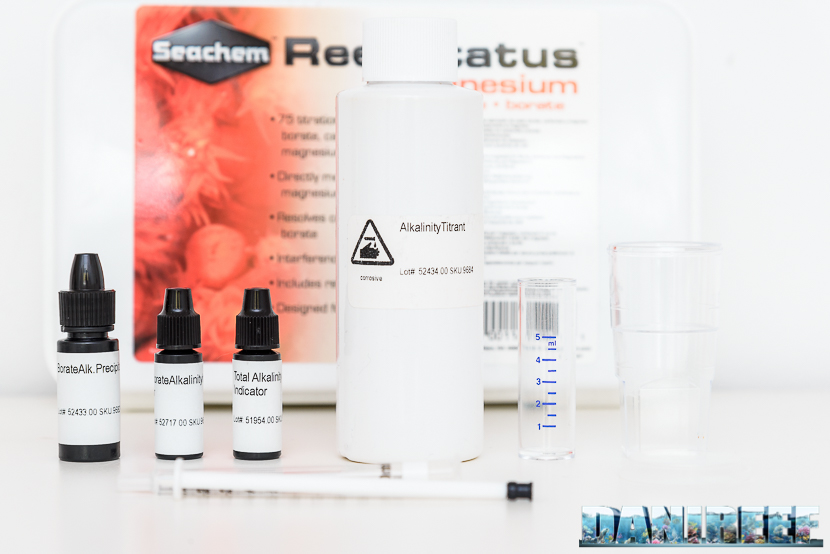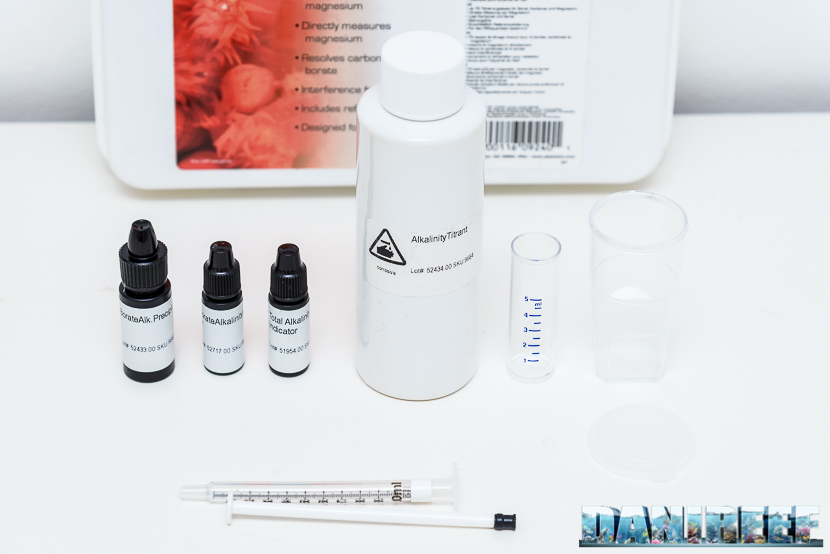# Seachem ReefStatus Magnesium Carbonate Borate test kit – Review

## Total alkalinity test

Alkalinity test are divided in in total alkalinity, borate alkalinity and carbonate alkalinity. Test is very simple and compared with the magnesium one you can make it quickly.

Fit 5 ml water sample to analyze inside the vial of observation, using the simple measurement vial, of course you may also  use a syringe for this, as I prefer to do.

Add one drop of Total Alkalinity Indicator and shake to mix the content.Fill the syringe up to the value of  1 ml, with the solution called “alkalinity titrant“, then start to dose drop by drop (titration), until the solution will pass from blue (given by the previous reagents) to yellow. It is suggested to shake after every drop to allow the melt solution.

Now every tenth of a milliliter corresponds to 1 meq/l. Every hundredth of a milliliter, or each notch, would correspond to about 0,1 meq/l, with the possibility of estimating by eye even lower values.

If the value of the total alkalinity is greater than 2,50 meq/l, or when you are going to use all the liquid in the syringe, you will have to refill the syringe and then add up the values provided.

We will have some problem because normally we used to calculate with german degree, where a 1 meq/l value about 2,8 dKH. So using a whole syringe we will have 2,5 meq/l or about 2,5*2,8=7 (you can help with this conversion tool ).## Borate Alkalinity test

Fill 5 ml sample of the water to analyze into the vial, using the simple measurement vial or a syringe as we told before.

Add two drops of Borate Alkalinity Precipitant and shake to mix the content. Then leave it for 5 minutes and then add a drop of Borate Alkalinity Indicator.

Fill the syringe up to the value of 1 ml, with the solution called  “alkalinity titrant“, then start to dose drop by drop (titration), until the solution will pass from blue color (given by the previous reagents) to yellow. It is suggested to shake after every drop to allow the melt solution. When you get to the blue-purple color you will need to add only a further drop.

Now every tenth of a milliliter corresponds to  1 meq/l. Every hundredth of a milliliter, or each notch, would correspond to about 0,1 meq/l, with the possibility of estimating by eye even lower values.

We will have some problem because normally we used to calculate with french degree, where a 1 meq/l value about 2,8 dKH. So using a whole syringe we will have 2,5 meq/l or about 2,5*2,8=7 (you can help with this conversion tool ).

Normally the borate alkalinity is about one tenth of the total one, then about, 0,1/0,2 meq/l. Just to settling with the values at stake.

## Carbonate Alkalinity test

Simply subtract the Borate Alkalinity to the total one to have the Carbonate Alkalinity.

Final Thoughts

The test is not cheap it costs 65,00 euro, but the price is justified by the overall accuracy, as Fauna Marin and Triton measured as well.

Overall Score 4.5